Courses

# Test: Potential Energy

## 20 Questions MCQ Test Physics Class 11 | Test: Potential Energy

Description
This mock test of Test: Potential Energy for JEE helps you for every JEE entrance exam. This contains 20 Multiple Choice Questions for JEE Test: Potential Energy (mcq) to study with solutions a complete question bank. The solved questions answers in this Test: Potential Energy quiz give you a good mix of easy questions and tough questions. JEE students definitely take this Test: Potential Energy exercise for a better result in the exam. You can find other Test: Potential Energy extra questions, long questions & short questions for JEE on EduRev as well by searching above.
QUESTION: 1

### How much work is done by a man weighing 80 kgf in raising a stone of weight 20 kgf to the top of a building of height 40 m? (g = 9.8 m/s2)

Solution:

Total mass=80+20=100
w=mgh(where m=100, g=9.8, h=40m)
w=100×9.8×40
w=39200J
work done by man weighing 80 kg raising a stone of 20kgf to the top of building of Height is 40m is 39,200J

QUESTION: 2

### The potential energy of a body at height h is mgh. Then its kinetic energy just before hitting the ground is

Solution:

From the law of conservation of mechanical energy, the potential energy of a body at height h is converted into kinetic energy when it falls down. So, the magnitude of kinetic energy just before hitting the ground is equal to the potential energy at height h which is mgh.

QUESTION: 3

### When does the potential energy of a spring increase?

Solution:

Potential energy of a spring is proportional to the square of the difference of the springs length and its original length, hence whether it is compressed or stretched the potential the potential energy will eventually increase only.

QUESTION: 4

In the given figure, what is the work done by the spring force?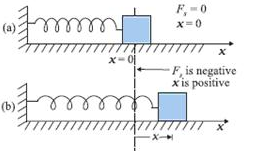Solution:

We know that for a spring with spring constant k, work done by the spring when a compression or expansion is done of some length say x is ½ kx2
Also as the work done is in the opposite direction to that of force applied, we get the sign of the force to be negative.

QUESTION: 5

Which of the following force is non-conservative

Solution:

Force between two stationary mass is gravitational force which is conservative. between two charge is electrostatic. muscular force is non conservative because we did work it lost or appear in form of heat and we need to eat food to gain energy for work done.

QUESTION: 6

A body dropped from height H reaches the ground with a speed of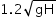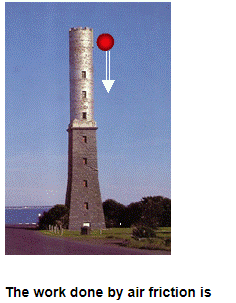Solution:

The forces acting on the body are the force of gravity and the air friction. By work energy theorem, the total work done on the body is
W=1/2m(1.2√gH)2−0=0.72mgH
The work done by the force of gravity is mgH.
. Hence, the work done by the air friction is,
=0.72mgH-mgH
=00.28mgH.

QUESTION: 7

You are in a lift moving from the 3rd floor to the 12th floor, through a height H. If the elevator moves at a constant speed without stopping, what is the work performed on you by the elevator? Take your body mass as M.

Solution:

According to Newton's third law of motion, the force exerted on the floor by the person is equal and opposite the force exerted on the person by the floor.
According to the second law of Newton's law of motion, the net force exerted on the person is equal to the person’s mass times the person’s acceleration.
The net force exerted on the person = Force exerted by the floor+ Force exerted by gravity.
If the elevator goes up with acceleration g, the same is the person inside the elevator.
The work done on the man by the floor is the sum of the change in potential energy and kinetic
energy.
i.e W = Δ P.E + Δ K.E
But the elevator moves at a constant speed, so K.E =0.
W = Δ P.E
The work performed on you by the elevator is force times displacement = -Mgh
Negative sign shows it is moving upwards, i.e., against g.

QUESTION: 8

An astronomer has total weight of 80 kg. On reaching moon, he climbs up 10 m to enter his aircraft by the ladder. His gravitational potential energy is increased by 1000 J. The value of the gravitational acceleration on the moon is

Solution:
QUESTION: 9

Out of a pair of identical springs of spring constants 240 N/m, one is compressed by 10 cm and other is stretched by 10 cm. The difference in P.E. stored in the two springs is:

Solution:

P.E. stored in a spring = 1/2Kx2
where x is the extension or compression. In both cases, work is done on the spring.
∴ For the same x, the same potential energy will be stored in the springs.
∴ The difference between the energies is zero.

QUESTION: 10

What is the dimension of k/m where k is the force constant and m is the mass of the oscillating object?

Solution:

F=k x (compression in string).
X=L.
a=LT-2.
F=ma.
ma= k L.
LT-2/L=k/m.
T-2=k/m.

QUESTION: 11

A ball of mass 2 kg is raised to the height of 60 m and then dropped from the top. In this case, the quantity which has different magnitude from the other given quantities is

Solution:

We know that the total work done by gravity is always equal to the gravitational force in magnitude as it is a conservative force and as because no other non conservative force acts upon the body we can say the mechanical energy remains conserved. Thus we get the potential energy at top is exactly equal to KE just before the ball hitting the ground.

QUESTION: 12

A hawk of mass 10 kg dives to catch a mouse. During dive it loses 500 J of its potential energy. The height from where it begins its dive is (take g = 10 m/s2)

Solution:

Change in GPE (Gravitational Potential energy) = mass x gravity x change in height
500 = 10 x 10 x change in height

500 / 100 = change in height

So, height from where it begins its dive = 5 m

QUESTION: 13

A vehicle of 50 quintal climbs up a hill of 10 m. The potential energy gained by it is (take g = 10 m/s2)

Solution:

mass = 50 x 100 = 5000 kg, g = 10 m/s2,  h = 10 m
Potential energy gained = mgh = 5000 x 10 x 10 = 5 x 105 J

QUESTION: 14

Select the odd one out

Solution:

In A stretched bow. Compressed gas, compressed or elongated spring there is elastic potential energy but the lifted weight there is gravitational force, so the odd one here is lifted weight.

QUESTION: 15

You are in a lift moving from the 3rd floor to the 12th floor, having a height difference of H = 40 m. Your mass is M = 80 kg and the acceleration due to gravity is g = 10 m/s2. If the elevator moves at a constant speed without stopping, what is the total work performed by all of the forces together (the net force) acting on you?

Solution:

As the final velocity after the action of all forces is 0, therefore the person has 0 net acceleration.

And, as we know that

Fnet = m.anet

Therefore, the net force is 0 and hence, the net work done is also 0.

QUESTION: 16

An elastic spring of force constant k is compressed by an amount x. Then its potential energy will be

Solution: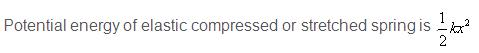QUESTION: 17

A plot of potential energy and kinetic energy versus displacement x for a block attached to a spring obeying Hooke’s law is

Solution:

The graphs are parabolas as the potential and kinetic energy vary as x2.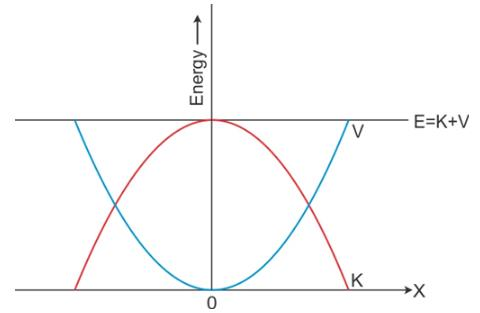QUESTION: 18

The principle of conservation of energy is represented by

Solution:

The law of conservation of energy states that: the total mechanical energy of a system remains unchanged (constant) in any isolated system of objects that interact only through conservative forces. In other words, it states that: the energy may neither be created nor destroyed, but transferred from a form of energy to another. The total mechanical energy, E, is defined as:[ E = K + U] Where K is the total kinetic energy and U is the total potential energy of the system.

The conservation law of energy is , therefore, [Ei = Ef ],Where Ei and Ef are the initial and final energies, respectively. That implies to Ki + Ui = Kf + Uf

QUESTION: 19

Which of the following is not a kind of potential energy?

Solution:

Potential Energy is any type of stored energy. It can be chemical, nuclear, gravitational, or mechanical. Kinetic Energy is found in movement. Nuclear power plants change the nuclear potential energy of uranium or plutonium into electricity too.

QUESTION: 20

For a varying force work done can be expressed as a

Solution:

For a variable force, the work done can be expressed as a definite integral of force over displacement for any system.In case of a variable force, the is calculated with the help of integration. For example, in the case of a spring, the force acting upon any object attached to a horizontal spring can be given as Fs = -kx, where k is the spring constant and x is the displacement of the object attached. We can see that this force is proportional to the displacement of the object from the equilibrium position, hence the force acting at each instant during the compression and extension of the spring will be different. Thus, the infinitesimally small contributions of work done during each instant are to be counted in order to calculate the total work done.